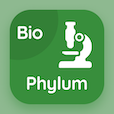Colleges Online Courses

Applied Physics Quizzes

Applied Physics Quiz PDF - Complete

# Product of Two Vectors Multiple Choice Questions p. 50

Study Product of Two Vectors multiple choice questions and answers, product of two vectors quiz answers PDF 50 to study Applied Physics course online. Vector and Equilibrium MCQ trivia questions, product of two vectors Multiple Choice Questions (MCQ) for online college degrees. "Product of Two Vectors MCQ" PDF eBook: viscosity of liquids, angular and linear velocities, applied physics: physical quantities, cathode ray oscilloscope, product of two vectors test prep for best two year degrees.

"Multiplication of two vectors is named as" MCQ PDF: vector multiplication, scalar multiplication, point multiplication, and both a and b for online college courses. Learn vector and equilibrium questions and answers to improve problem solving skills for GRE subject tests.

## Product of Two Vectors Questions and Answers MCQs

MCQ: Multiplication of two vectors is named as

scalar multiplication
vector multiplication
point multiplication
both a and b

MCQ: The cathode ray oscilloscope displays graph of waveforms based on

current
voltage
potential difference
temperature

MCQ: Physical quantities that cannot be described with the help of other quantities are called

base quantities
derived quantities
mechanical quantities
both a and b

MCQ: In a spaceship, objects revolving around the Earth appears to be

small
large
weightless
massive

MCQ: At 30 °C, glycerin has viscosity of

2.801
4
1.9
6.29

### More Quizzes from Applied Physics Course